# Texas Go Math Grade 5 Lesson 8.3 Answer Key Multiplication and Division Equations

Refer to our Texas Go Math Grade 5 Answer Key Pdf to score good marks in the exams. Test yourself by practicing the problems from Texas Go Math Grade 5 Lesson 8.3 Answer Key Multiplication and Division Equations.

## Texas Go Math Grade 5 Lesson 8.3 Answer Key Multiplication and Division Equations

Unlock the Problem

Jake is buying a movie ticket and a box of popcorn for $12. The ticket costs 2 times as much as the popcorn. How much does the popcorn cost? How much does the ticket cost? The cost of the tickets is 2 × p, where p is the price of the popcorn. • How does knowing that a ticket costs 2 times as much as the popcorn help you choose an operation to write an expression? ________________________ The expression is 2 x p + p =$12

Use a related equation.

STEP 1:
Complete the strip diagram to represent the popcorn and ticket cost.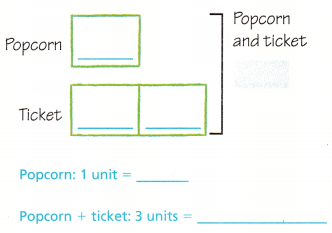The popcorn costs p. Label the strip for the popcorn.
It represents 1 unit.
The cost of the ticket is _________ times as much. Label the strips for the ticket.
What is the total cost? ___________
How many units are shown in the model? __________

STEP 2:

Use the strip diagram to write a multiplication equation.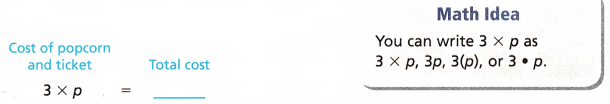To find a missing factor, write a division equation that is related to the multiplication equation. Then divide.
________ ÷ 3 = p __________ = p
So, 1 bag of popcorn costs ___________.
The cost 1 tickets is 2 times the cost of 1 bag of popcorn.
So, 1 ticket costs _________.
STEP 1:The popcorn costs p is $4. Label the strip for the popcorn. It represents 1 unit. The cost of the ticket is 2 x p times as much. Label the strips for the ticket. The total cost is$12.
3 units are shown in the model.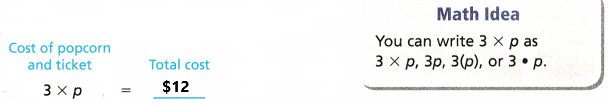To find a missing factor, We have to write a division equation that is related to the multiplication equation. Then divide.
12 ÷ 3 = p
4 = p
So, 1 bag of popcorn costs  $4. The cost 1 tickets is 2 times the cost of 1 bag of popcorn. 2 x$4 = $8 So, 1 ticket costs$8.

Example

Use a related equation.
Katrina buys a package of trading cards. She divides the cards into 4 equal piles. Each pile contains 12 cards. The equation that describes the number of cards in the package is c ÷ 4 = 12. How many cards were in the package when Katrina bought it?

Write a related equation.
MODEL
• Use a strip diagram to represent the problem.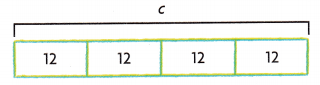SOLVE
Write a related multiplication equation.
Multiplication equation: _____________
c = ____________
c ÷ 4 = 12
Multiplication equation:
c = 12 x 4
c = 48
Explanation:
Katrina buys a package of trading cards. She divides the cards into 4 equal piles. Each pile contains 12 cards. The equation that describes the number of cards in the package is c ÷ 4 = 12. The multiplication equation is c = 12 x 4
the product is 48. So the value of c is 48. So, the package Katrina bought had 48 trading cards in it.

Math Talk
Mathematical Processes

Explain how the strip diagrams for multiplication and division are related.

Share and Show

Use the strip diagrams to write an equation. Then solve.

Question 1.
The cost of 3 pencils is 96 cents. Each pencil costs the same. What is the cost of 1 pencil, p?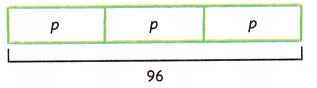Equation: _______ = 96 p = __________
One pencil costs __________ cents.Equation:
3 x p = 96
p = 96 ÷ 3
p = 32
One pencil costs 32 cents.
Explanation:
The cost of 3 pencils is 96 cents. Each pencil costs the same. The equation is 3 x p = 96. To calculate the p value we have to perform division operation. Divide 96 by 3 the quotient is 32. The value of p is 32. One pencil costs 32 cents.

A gardener is planting 6 rows of spring bulbs. Each row has 15 bulbs. How many bulbs is he planting? Let b equal the total number of bulbs.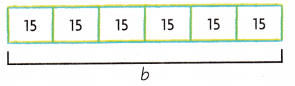Equation: ____________ b = ________
He is planting ________ bulbs.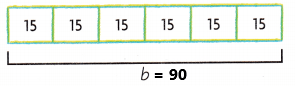Equation:
b ÷ 6 = 15
b = 15 x 6
b = 90
Here b is equal to the total number of bulbs.
He is planting 90 bulbs.
Explanation:
A gardener is planting 6 rows of spring bulbs. Each row has 15 bulbs. The equation is b ÷ 6 = 15. Perform multiplication operation to find the value of b. Multiply 15 with 6 the product is 90. Here b is equal to the total number of bulbs. He is planting 90 bulbs.

Problem Solving

Practice: Copy and Solve Use a strip diagram or a related equation to solve. Check your solution.

Question 3.
128 = 8 × d
128 = 8 × d
Related equation:
128 ÷  8 = d
16 = d
Explanation:
The given equation is 128 = 8 x d. We have to find out the d value. So, the related equation for the given equation is 128 ÷  8 = d. Perform division operation on 128 ÷  8 the quotient is 16. The value of d is 16.

Question 4.
r ÷ 9 = 17
r ÷ 9 = 17
Related equation:
r = 17 x 9
r = 153
Explanation:
The given equation is r ÷ 9 = 17. We have to find out the r value. So, the related equation for the given equation is r = 17 x 9. Perform multiplication operation with 17 and 9 the product is 153. The value of r is 153.

Question 5.
6m = 78
6m = 78
Related equation:
m = 78 ÷ 6
m = 13
Explanation:
The given equation is 6m = 78. We have to find out the m value. So, the related equation for the given equation is 78 ÷  6 = m. Perform division operation on 78 ÷  6 the quotient is 13. The value of m is 13.

Go Math 5th Grade Multiplication Equation Lesson 8.3 Question 6.
7 = b ÷ 17
7 = b ÷ 17
Related equation:
7 x 17 = b
119 = b
Explanation:
The given equation is 7 = b ÷ 17. We have to find out the b value. So, the related equation for the given equation is b = 7 x 17. Perform multiplication operation with 17 and 7 the product is 119. The value of b is 119.

Question 7.
How can you justify that j = 348 is the solution to j ÷ 12 = 29? Explain.
The equation is j ÷ 12 = 29
we know that j = 348
Related equation
j = 29 x 12
j = 348
The value j = 348 is the solution to j ÷ 12 = 29.
Explanation:
The given equation is j ÷ 12 = 29. Here j is equal to 348. So, the related equation for the given equation is
j = 29 x 12 Perform multiplication operation with 29 and 12 the product is 348. Hence the value j is equal to 348 is the solution to j ÷ 12 = 29.

Problem Solving

Use the table to complete 8-9. Use a strip diagram to write each equation. Then solve.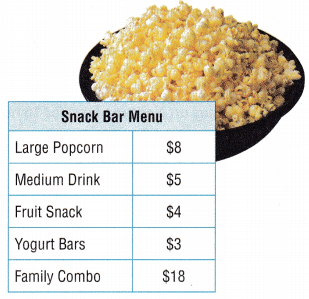Question 8.
Multi-Step On Friday, the snack bar made $992 selling large buckets of popcorn. How many large buckets of popcorn did the snack bar sell on Friday? In your equation1 let p represent the number of large buckets of popcorn sold. Answer: Question 9. H.O.T. Multi-Step On Thursday, the snack bar made total of$340 in medium drink sales and a total of $216 in yogurt bar sales. Which item did the snack bar sell more of on Thursday? Explain how you found your answer.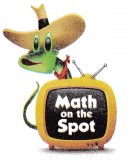Answer: Question 10. H.O.T. Multi-Step Asher wants to buy a video game console. In order to save the money needed to buy it in 5 months, he divides the cost by 5. He finds he needs to save$37 a month. What is the total cost, c, of the game console?
Equation:
c ÷ 5 = $37 Related equation: c =$37 x 5
c = $185 The total cost of the video game console is$185.
Explanation:
Asher wants to buy a video game console. In order to save the money needed to buy it in 5 months, he divides the cost by 5. He finds he needs to save $37 a month. The equation is c ÷ 5 =$37. The related equation for the given equation is c = $37 x 5. Perform multiplication operation with 37 and 5 the product is$185. The total cost of the video game console is $185. Go Math Grade 5 Lesson 8.3 Answer Key Question 11. Representations Mariah bought a bag of buttons. She divides the buttons equally among 15 containers. Each container has 10 buttons. How many buttons does Mariah have? In your equation, let b represent the number of buttons Mariah has. Answer: Equation: b ÷ 15 = 10 Related equation: b = 10 x 15 b = 150 Here b represents the number of buttons does Mariah have. Mariah have 150 buttons. Explanation: Mariah bought a bag of buttons. She divides the buttons equally among 15 containers. Each container has 10 buttons. The equation is b ÷ 15 = 10. The related equation for the given equation is b = 10 x 15. Perform multiplication operation with 10 and 15 the product is 150. Here b represents the number of buttons does Mariah have. Mariah have 150 buttons. Daily Assessment Task Fill in the bubble completely to show your answer. Question 12. Mr. Tune took a vacation to Neptune. His vacation lasted for 144 hours. A day on Neptune lasts for 16 hours. Which equation and solution shows how many days Mr. Tune’s vacation lasted? (A) 16d = 144; d = 9 (B) d = 16 × 144; d = 2,304 (C) 16 + d = 144; d = 128 (D) d = 16 + 144; d = 160 Answer:Equation: 16 x d = 144 d = 144 ÷ 16 d = 9 Mr. Tune’s vacation lasted for 9 days. So, option A is correct. Explanation: Mr. Tune took a vacation to Neptune. His vacation lasted for 144 hours. A day on Neptune lasts for 16 hours. The equation is 16 x d = 144. The related equation for this equation is d = 144 ÷ 16. Divide 144 by 16 the quotient is 9. Mr. Tune’s vacation lasted for 9 days. Question 13. Which equation does the strip diagram represent?(A) 6a = 12 (b) a ÷ 6 = 12 (C) 12a = 6 (D) a + 6 = 12 Answer:a ÷ 6 = 12 a = 12 x 6 a = 72 So, option B is correct Explanation: For the above strip diagram, we can observe that each block has 12 and there are a total of 6 blocks. The equation is a ÷ 6 = 12. The related equation for this equation is a = 12 x 6. Perform multiplication operations on 12 and 6 the product is 72. So, option B is correct. Go Math Division Equations Grade 5 Lesson 8.3 Question 14. Multi-Step Noah bought 5 CDs and a DVD for a total of$57. The DVD cost $12. The CDs were each the same price. How much did Noah pay for each CD? (A)$12
(B) $15 (C)$11
(D) $9 Answer: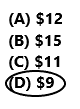5 CDs + DVD =$57
5 CDs + $12 =$57
5 CDs = $57 –$12
5 CDs = $45 1 CD =$45 ÷ 5 = $9 Noah pay$9 for each CD.
So, option D is correct.
Explanation:
Noah bought 5 CDs and a DVD for a total of $57. The DVD cost$12. The CDs were each the same price. The equation is 5 CDs + $12 =$57. Subtract $12 from$57 the difference is $45. Divide$45 by 5 the result is $9. Noah pay$9 for each CD. So, option D is correct.

Texas Test Prep

Question 15.
Rick earned $133 for 7 hours of work. How much does Rick earn per hour? The equation 7p = 133 can be used to find p, the amount Rick earns per hour. (A)$19
(B) $6 (C)$931
(D) $69 Answer:7p =$133
p = $133 ÷ 7 p =$19
Rick earns $19 per hour. So, option A is correct. Explanation: Rick earned$133 for 7 hours of work. The equation 7p = 133 can be used to find p, the amount Rick earns per hour is calculated by dividing 133 by 7 the result is 19. Rick earns $19 per hour. So, option A is correct. ### Texas Go Math Grade 5 Lesson 8.3 Homework and Practice Answer Key Use a strip diagram or a related equation to solve. Check your solution. Question 1. c ÷ 5 = 13 Answer: c ÷ 5 = 13 Related equation: c = 13 x 5 c = 65 Explanation: The given equation is c ÷ 5 = 13. We have to find out the c value. So, the related equation for the given equation is c = 13 x 5. Perform multiplication operation with 13 and 5 the product is 65. The value of c is 65. Question 2. 112 = 7 × b Answer: 112 = 7 × b Related equation: 112 ÷ 7 = b 16 = b Explanation: The given equation is 112 = 7 x b. We have to find out the b value. So, the related equation for the given equation is 112 ÷ 7 = b . Perform division operation 112 by 7 the result is 16. The value of b is 16. Texas Go Math Practice and Homework Lesson 8.3 Answer Key Question 3. 4p = 68 Answer: 4p = 68 Related equation: p = 68 ÷ 4 p = 17 Explanation: The given equation is 4p = 68. We have to find out the p value. So, the related equation for the given equation is 68 ÷ 4 =p . Perform division operation on 68 by 4 the result is 17. The value of p is 17. Question 4. 9 = d ÷ 21 Answer: 9 = d ÷ 21 Related equation: 9 x 21 = d 189 = d Explanation: The given equation is 9 = d ÷ 21. We have to find out the d value. So, the related equation for the given equation is d = 9 x 21. Perform multiplication operation with 9 and 21 the product is 189. The value of d is 189. Question 5. 105 = 3 × a Answer: 105 = 3 x a Related equation: 105 ÷ 3 = a 35 = a Explanation: The given equation is 105 = 3 x a. We have to find out the a value. So, the related equation for the given equation is 105 ÷ 3 =a . Perform division operation on 105 by 3 the result is 35. The value of a is 35. Question 6. 9g = 99 Answer: 9g = 99 Related equation: g = 99 ÷ 9 g = 11 Explanation: The given equation is 9g = 99. We have to find out the g value. So, the related equation for the given equation is g = 99 ÷ 9. Perform division operation on 99 by 9 the result is 11. The value of g is 11. Question 7. m ÷ 10 = 16 Answer: m ÷ 10 = 16 Related equation: m = 16 x 10 m = 160 Explanation: The given equation is m ÷ 10 = 16. We have to find out the m value. So, the related equation for the given equation is m = 16 x 10. Perform multiplication operations with 16 and 10 the product is 160. The value of m is 160. Go Math Lesson 8.3 5th Grade Division Answer Key Question 8. 22 = n ÷ 7 Answer: 22 = n ÷ 7 Related equation: 22 x 7 = n 154 = n Explanation: The given equation is 22 = n ÷ 7. We have to find out the n value. So, the related equation for the given equation is n = 22 x 7. Perform multiplication operation with 22 and 7 the product is 154. The value of n is 154. Question 9. 92 = f × 4 Answer: 92 = f x 4 Related equation: 92 ÷ 4 = f 23 = f Explanation: The given equation is 92 = f x 4. We have to find out the f value. So, the related equation for the given equation is f = 92 ÷ 4. Perform division operation on 92 by 4 the result is 23. The value of f is 23. Question 10. h ÷ 9 = 14 Answer: h ÷ 9 = 14 Related equation: h = 14 x 9 h = 126 Explanation: The given equation is h ÷ 9 = 14. We have to find out the h value. So, the related equation for the given equation is h = 14 x 9. Perform multiplication operation with 14 and 9 the product is 126. The value of h is 126. Question 11. 13 = j ÷ 8 Answer: 13 = j ÷ 8 Related equation: 13 x 8 = j 104 = j Explanation: The given equation is 13 = j ÷ 8. We have to find out the j value. So, the related equation for the given equation is 13 x 8 = j. Perform multiplication operation with 13 and 8 the product is 104. The value of j is 104. Question 12. 165 = 11r Answer: 165 = 11r Related equation: 165 ÷ 11 = r 15 = r Explanation: The given equation is 165 = 11r. We have to find out the r value. So, the related equation for the given equation is r = 165 ÷ 11 . Perform division operation on 165 by 11 the result is 15. The value of r is 15. Problem Solving Question 13. Sierra arranges fabric squares to sew together to make a blanket for her baby sister. She divides the fabric squares equally among 12 rows. Each row has 8 squares. How many fabric squares does Sierra have? In your equation, let f represent the number of fabric squares Sierra has. Answer: f ÷ 12 = 8 Related equation: f = 8 x 12 f = 96 Here f represent the number of fabric squares does Sierra have. Sierra have 96 fabric squares. Explanation: Sierra arranges fabric squares to sew together to make a blanket for her baby sister. She divides the fabric squares equally among 12 rows. Each row has 8 squares. The equation is f ÷ 12 = 8. The related equation is f = 8 x 12. Multiply 8 with 12 the product is 96. Here f represent the number of fabric squares. Sierra have 96 fabric squares. Go Math Grade 5 Lesson 8.3 Division Strip Diagrams Question 14. A school district purchased 90 new computers. The computers were divided equally among 6 classrooms. How many new computers are in each classroom? In your equation, let c represent the number of computers in each classroom. Answer: 90 ÷ 6 = c 15 = c Here c represent the number of computers in each classroom. In each classroom there are 15 computers. Explanation: A school district purchased 90 new computers. The computers were divided equally among 6 classrooms. The equation is 90 ÷ 6 = c. Divide 90 by 6 the result is 15. Here c represent the number of computers in each classroom. In each classroom there are 15 computers. Question 15. Write a real-world problem for the equation 4 × y = 60. Then solve. Answer: Lesson Check Fill In the bubble completely to show your answer. Question 16. Which equation does the strip diagram represent?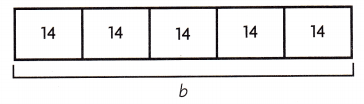(A) b ÷ 5 = 14 (B) 14b = 5 (C) 14 ÷ 5 = b (D) 5b = 14 Answer:b ÷ 5 = 14 b = 14 x 5 b = 70 So, option A is correct. Explanation: In the above strip diagram we can observe 5 rows with each row having 14. We have to write the equation by using strip diagram. The equation is b ÷ 5 = 14. The value b is calculated by multiplying 14 with 5 the product is 70. So, option A is correct. Question 17. Which equation does the strip diagram represent?(A) d + 4 = 88 (B) d ÷ 4 = 88 (C) 4d = 88 (D) d = 4 × 88 Answer:Option c is correct. Explanation: The above strip diagram represents the equation is 88 ÷ 4 = d. The related equation is 4d = 88. So option C is correct. Question 18. Dori earned 95 points on her science test. She received 5 points for each question she answered correctly. How many questions did Dori answer correctly? The equation 5q = 95 can be used to find q, the number of questions answered correctly. (A) 100 (B) 90 (C) 19 (D) 11 Answer:5q = 95 Related equation q = 95 ÷ 5 q = 19 Here q is the number of questions answered correctly. So, option C is correct. Explanation: Dori earned 95 points on her science test. She received 5 points for each question she answered correctly. The equation 5q = 95 can be used to find q, the number of questions answered correctly. Divide 95 by 5the result is 19. So, option C is correct. Question 19. The Adams family drove 270 miles to reach their vacation destination. If they drove 45 miles each hour, how many hours did they drive? Which equation can you use to represent the problem? (A) h = 270 × 45 (B) 270 = 45h (C) 45 ÷ h = 270 (D) h + 45 = 270 Answer: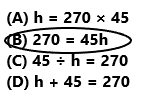Option B is correct. Explanation: The Adams family drove 270 miles to reach their vacation destination. If they drove 45 miles each hour then we have to calculate how many hours did they drive. The equation that represents the problem is 270 = 45 x h. So, option B is correct. Go Math Grade 5 Chapter 8 Lesson 8.3 Answer Key Question 20. Multi-Step The movie theater has 176 seats. There are 9 rows of 18 seats plus an additional section of handicapped-accessible seats. Write and solve an equation to find the number of handicapped-accessible seats in the theater. (A) 9 (B) 14 (C) 4 (D) 12 Answer:18 x 9 = 162 176 – 162 = 14 The number of handicapped-accessible seats in the theater is 14. So, option B is correct. Explanation: The movie theater has 176 seats. There are 9 rows of 18 seats plus an additional section of handicapped-accessible seats. Multiply 18 seats with 9 the product is 162. Subtract 162 from 176 the difference is 14. The number of handicapped-accessible seats in the theater is 14. So, option B is correct. Question 21. Multi-Step Sergei had$75 when he arrived at the mall. He spent $9 at the food court. Then he bought 3 posters that each cost the same amount. Sergei has$27 left. Write and solve an equation to find the cost of one poster.
(A) $9 (B)$3
(C) $13 (D)$7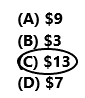He spent $9 at the food court.$75 – $9 – 3p =$27
$75 –$9 – $27 = 3p$39 = 3p
$39 ÷ 3 = p$13 = p
The cost of one poster is $13. So, option C is correct. Explanation: Sergei had$75 when he arrived at the mall. He spent $9 at the food court. Then he bought 3 posters that each cost the same amount. Sergei has$27 left. The equation is $75 –$9 – 3p = $27. Subtract$27 and $9 from$75 the difference is $39. Divide$39 ÷ 3 the result is $13. The cost of one poster is$13. So, option C is correct.